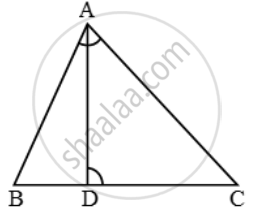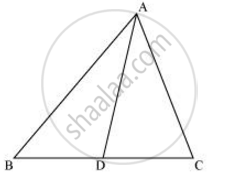# D is a point on the side BC of ∆ABC such that ∠ADC = ∠BAC. Prove that CA/CD=CB/CAor, CA^2 = CB × CD. - Mathematics

D is a point on the side BC of ∆ABC such that ∠ADC = ∠BAC. Prove that  \frac{CA}{CD}=\frac{CB}{CA} or, CA^2 = CB × CD.

#### Solution 1

In ∆ABC and ∆DAC, we have

∠ADC = ∠BAC and ∠C = ∠CTherefore, by AA-criterion of similarity, we have

∆ABC ~ ∆DAC

\Rightarrow \frac{AB}{DA}=\frac{BC}{AC}=\frac{AC}{DC}

\Rightarrow \frac{CB}{CA}=\frac{CA}{CD}

#### Solution 2∠ACD = ∠BCA (Common angle)

∴ ΔADC ∼ ΔBAC (By AA similarity criterion)

We know that corresponding sides of similar triangles are in proportion.

:. (CA)/(CB) = (CD)/(CA)

=> CA2 = CB x CD

Concept: Criteria for Similarity of Triangles
Is there an error in this question or solution?

#### APPEARS IN

NCERT Class 10 Maths
Chapter 6 Triangles
Exercise 6.3 | Q 13 | Page 141